### Quantum Computing

An algorithm is a step-by-step procedure for completing a specific task. In other words, it’s a way of doing something.

But to computer scientists, an algorithm is usually more than a procedure for completing a task; it is a procedure for solving a problem. Some problems are easy to solve: like addition, sorting a list, or finding the greatest common divisor of a set. But other problems are very difficult to solve; finding the prime factors of a large number is so hard that an entire field of public-key encryption is based on the assumption that it is practically impossible.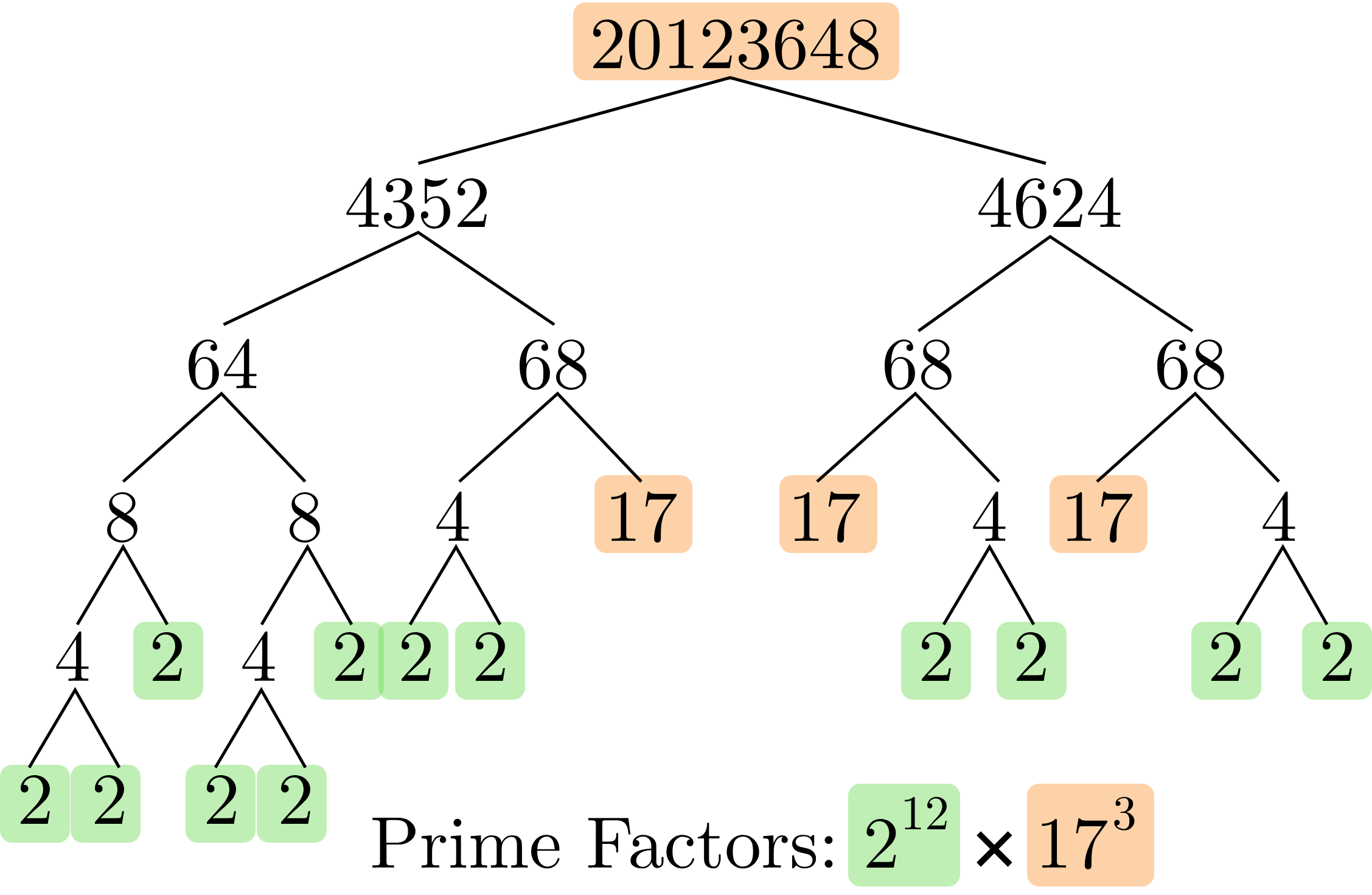In this quiz, we'll learn how a classical computer carries out a simple algorithm using bits and logical circuits.

# Computing with Circuits

While a recipe takes some ingredients as inputs and produces a dish as its output, a computer science algorithm takes data as input and produces different data as output.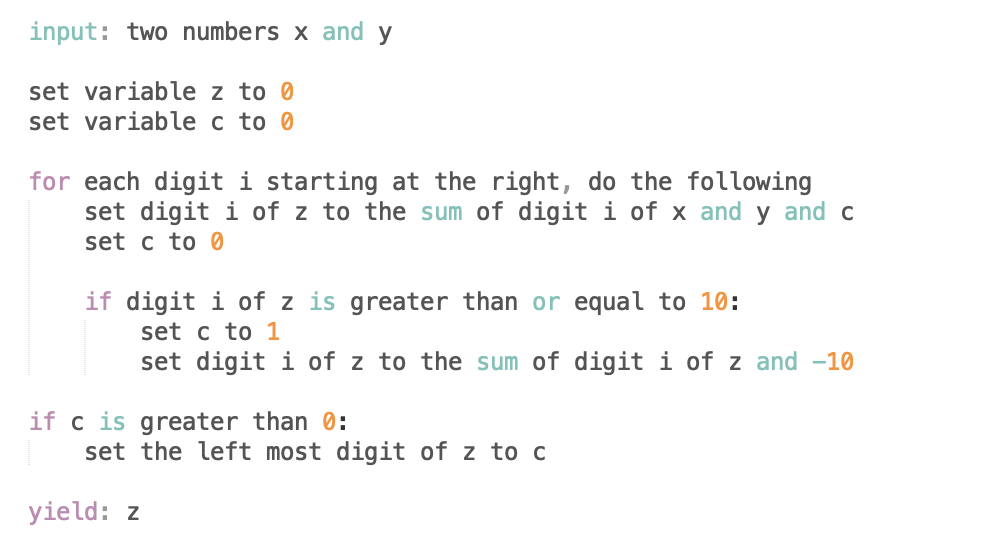The algorithm above states specific steps to be followed with the two numbers $x$ and $y.$

What does this algorithm output as $z?$

Note: that algorithm above could not be immediately run by a computer, because it's not written in a programming language. It's written in pseudocode, a sort of shorthand that maintains the explicit nature of computer code while being easy for humans to read.

# Computing with Circuits

In elementary school, you probably memorized sums of single-digit numbers: $4 + 7 = 11, \quad 3 + 6 = 9,\quad \textrm{ etc.}$ Later on, you learned a method for adding multiple-digit numbers, which was based on the single-digit additions that you had memorized. For example, you were asked to compute things like this: $\begin{array}{c} \phantom{+ 9}9384\\ \underline{ + \phantom{9}1456}\\ \phantom{ + } 10840\\ \end{array}$ The method you probably learned was the sequence of simple steps in the last pane, requiring repeated operations of the simple sums of single-digit numbers that you already memorized.

Can these steps be followed to add any two positive integers, no matter how many digits they have?

# Computing with Circuits

When humans write down algorithms for other humans to follow (like cooking recipes, or elementary school lesson plans), the instructions are usually given in a straightforward way that doesn’t waste time explaining things that should be commonly understood. The simple addition algorithm doesn't explain how to add two single-digit numbers, and a recipe doesn't explain how to crack an egg.

But when a human is specifying an algorithm to a computer, they need to be as precise as possible.

This is traditionally done using a programming language: a set of precise commands that a human can feed to a computer that a computer understands. This language serves as a tool that humans use to make it easier to communicate algorithms to a computer. At its heart, what a computer really understands as the specification of an algorithm is a binary logical circuit.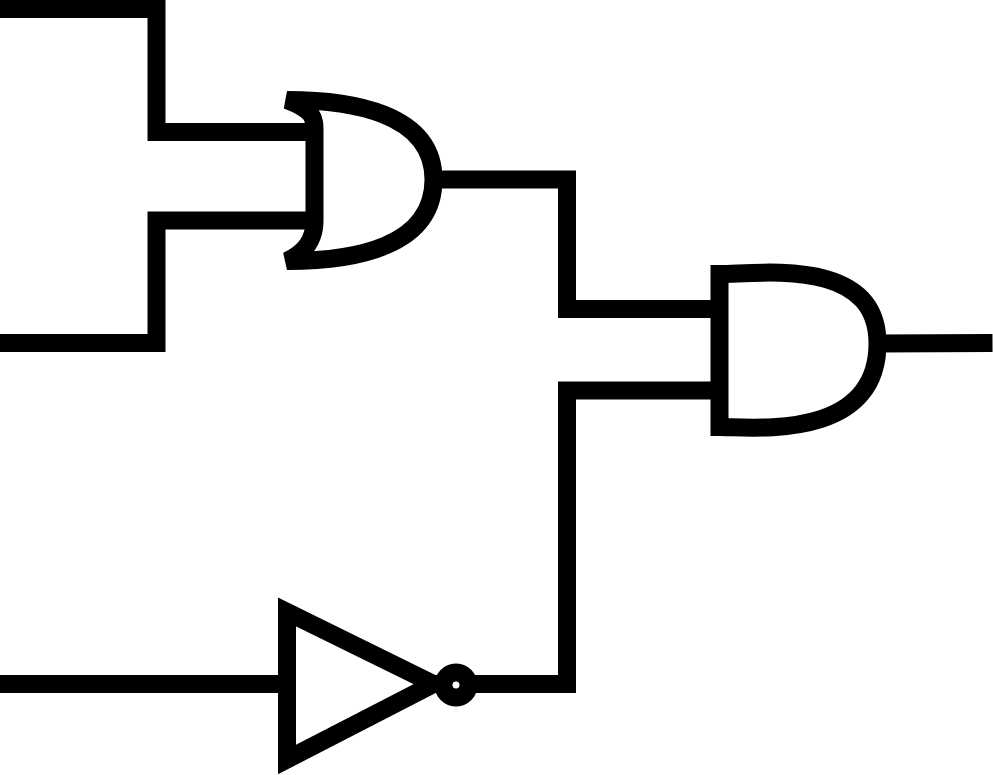# Computing with Circuits

Computers think in binary because the transistors that their processors are built from only have two states: $1$ and $0$.

Algorithms written in programming languages like C++ or Python as well as any strings or base-$10$ numbers involved are eventually translated into a simple set of instructions which operate on binary variables, long strings of $1$ and $0:$ \begin{aligned} \textrm{5728} & \rightarrow \tt{1011001100000} \\ \texttt{"Quantum"} & \rightarrow \tt{0111000101110101011000010110111\ldots} \end{aligned} A fundamental result in computer science showed that any problem can be solved using only simple operations on binary strings (or any other "alphabet" that has two or more symbols).

We generally think of adding two base-$10$ numbers as adding them one digit at a time, carrying the excess, and moving on to the next digit.

How could a computer adapt this to an algorithm to be performed on two binary strings $x$ and $y?$

# Computing with Circuits

Logical circuits are made up of gates that operate on bits. There's the $\mathbf{NOT}$ gate which flips a bit from $1$ to $0$, and the $\mathbf{AND}$ gate which takes in two bits as input and outputs $1$ if both inputs are $1,$ otherwise it outputs $0.$ You may have heard of many of these simple logical operations: $\mathbf{NAND},$ $\mathbf{OR},$ $\mathbf{XOR},$ etc.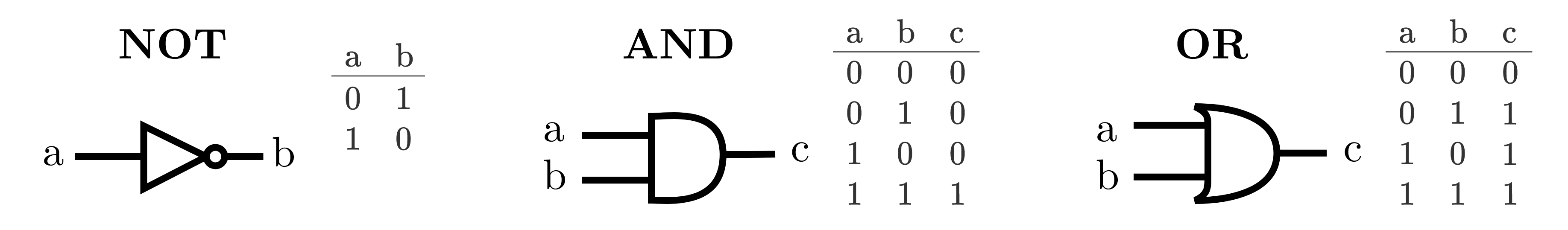Unfortunately, "adding" (the operation that's necessary to perform our algorithm) is not one of these basic operations. But, like any other computation, we can build an adding circuit using only these simple gates.

The adding logical circuit must have two input bits; $x$ and $y$. How many output bits does it need?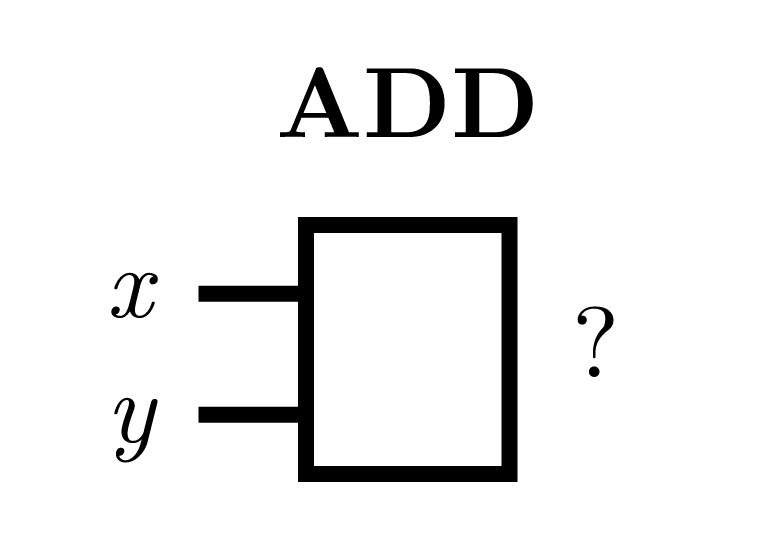# Computing with Circuits

If the inputs are $x = 1$ and $y = 1,$ then the (binary) sum is going to be $10,$ which requires two bits to represent. This is why we need a carry bit as well, just like the carrying variable from the elementary school addition algorithm.

The core of the binary adder is a gate called $\mathbf{XOR}$ (eXclusive OR), which will appear again and again in the quantum algorithms we will encounter later in this course.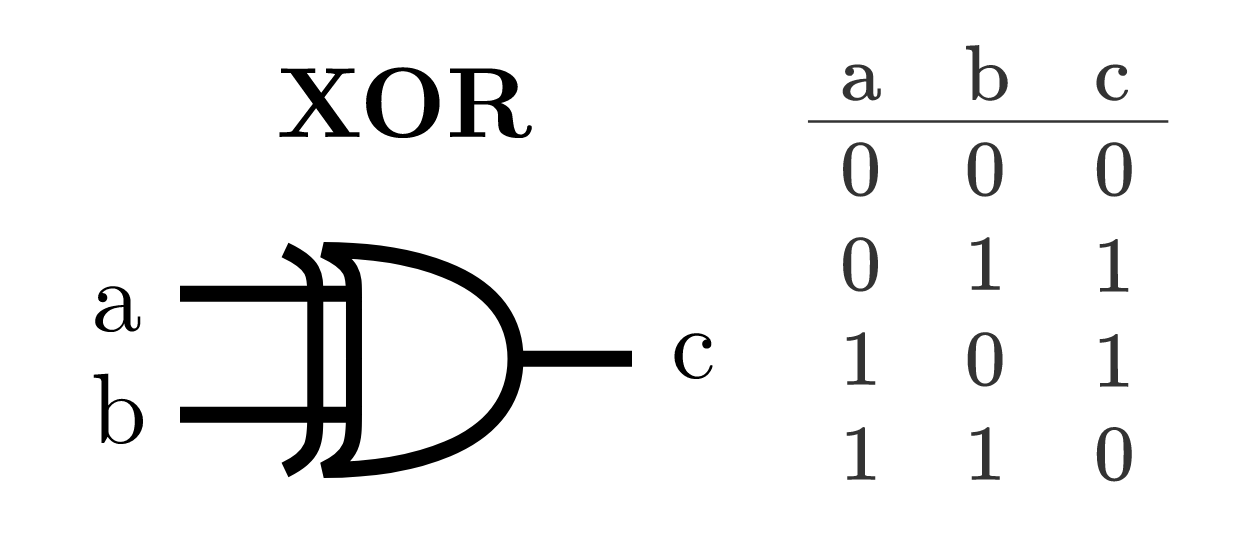The $\mathbf{XOR}$ computes $x \oplus y,$ given inputs $x$ and $y,$ where the $\oplus$ symbol implies binary addition, where the result is given modulo $2.$ This gate will always return the $2^0$ place of a sum given two binary inputs.

From inputs $x$ and $y,$ what gate can we use to determine the carry bit $c$ of the adding operation?

# Computing with Circuits

The resulting circuit we’ve constructed is called a “half-adder”: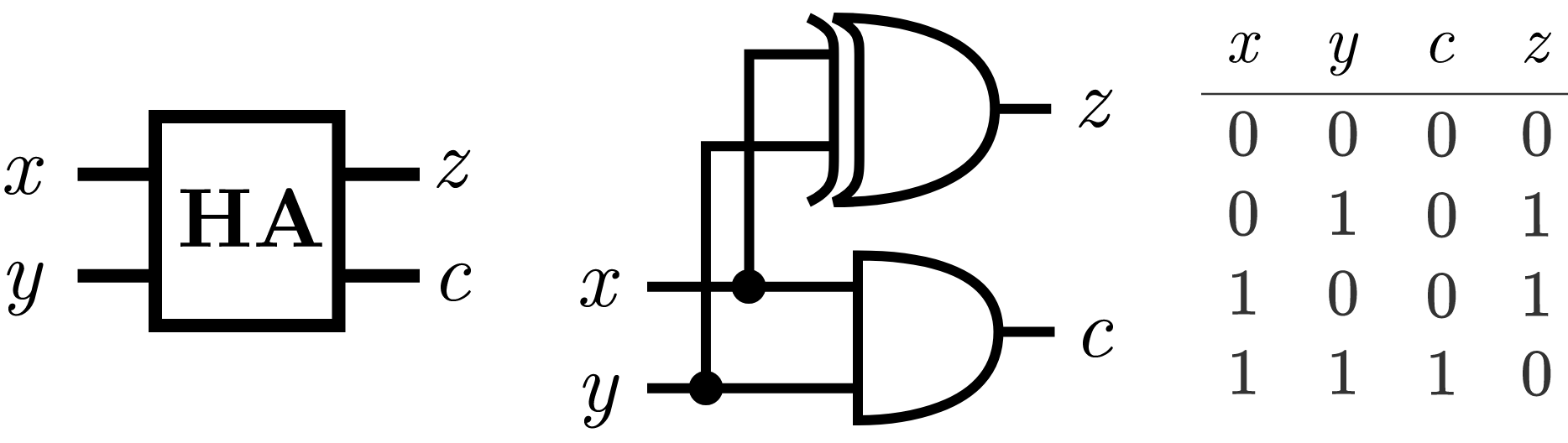But a half-adder isn't enough to carry out our adding algorithm. One step of the elementary school algorithm requires adding three binary bits: $x,$ $y,$ and $c.$

To do this, we need to cascade two half-adders in a row, to make the full adder below. This circuit is designed to be modular in a larger arithmetic operation — it takes in two binary inputs to be added, $x$ and $y,$ along with a carry bit $c$ that may have resulted from a previous adding operation.

It yields the sum of $z=x + y + c \pmod 2,$ along with a new carry bit, $c^\prime.$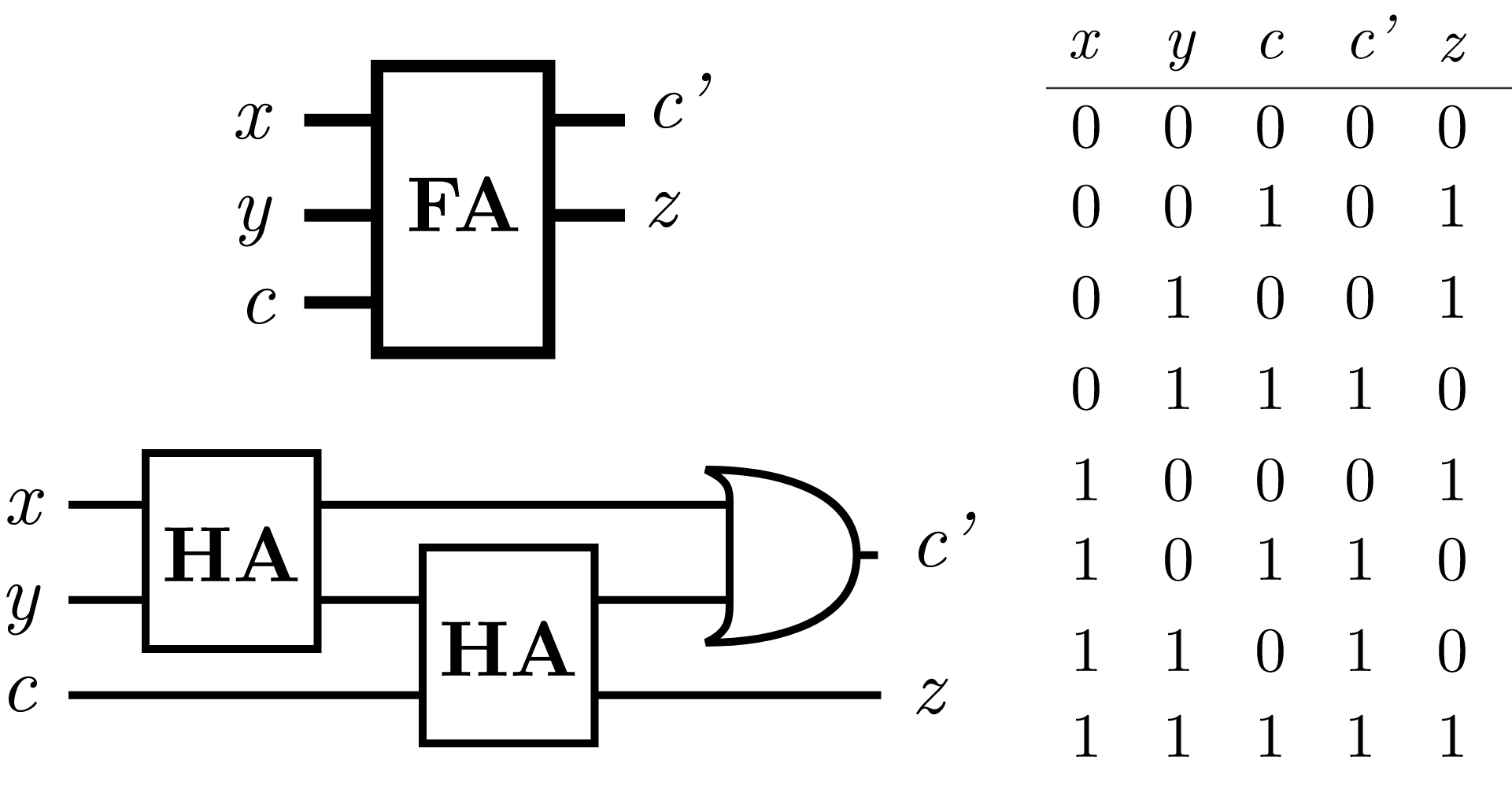# Computing with Circuits

We’ve found a construction of logical gates that we can box away into a convenient package that we call $\mathbf{FA}$, for “full-adder”.With this simple elementary school operation in our toolbox, we can walk through the pseudo-code algorithm and construct the equivalent circuit using the $\mathbf{FA}$ gate.

How many applications of $\mathbf{FA}$ are required to add together two $n$-bit binary numbers?

# Computing with Circuits

Adding two $n$-bit numbers requires $n$ repetitions of the black box computation we put together, just as the pseudo-code algorithm or elementary school addition requires $n$ single-digit additions to sum two $n$-digit numbers.

Let's say we'd like to use this algorithm to add $x = 15$ and $y = 12$. First, we need to convert them both to their binary (base-$2$) representations: \begin{aligned} 15_{10} &= 1111_{2} \\ 12_{10} &= 1100_{2}. \end{aligned} This summation would require 4 applications of the $\textrm{FA}$ operation, and yields $z = x+y = 11011 = 27$.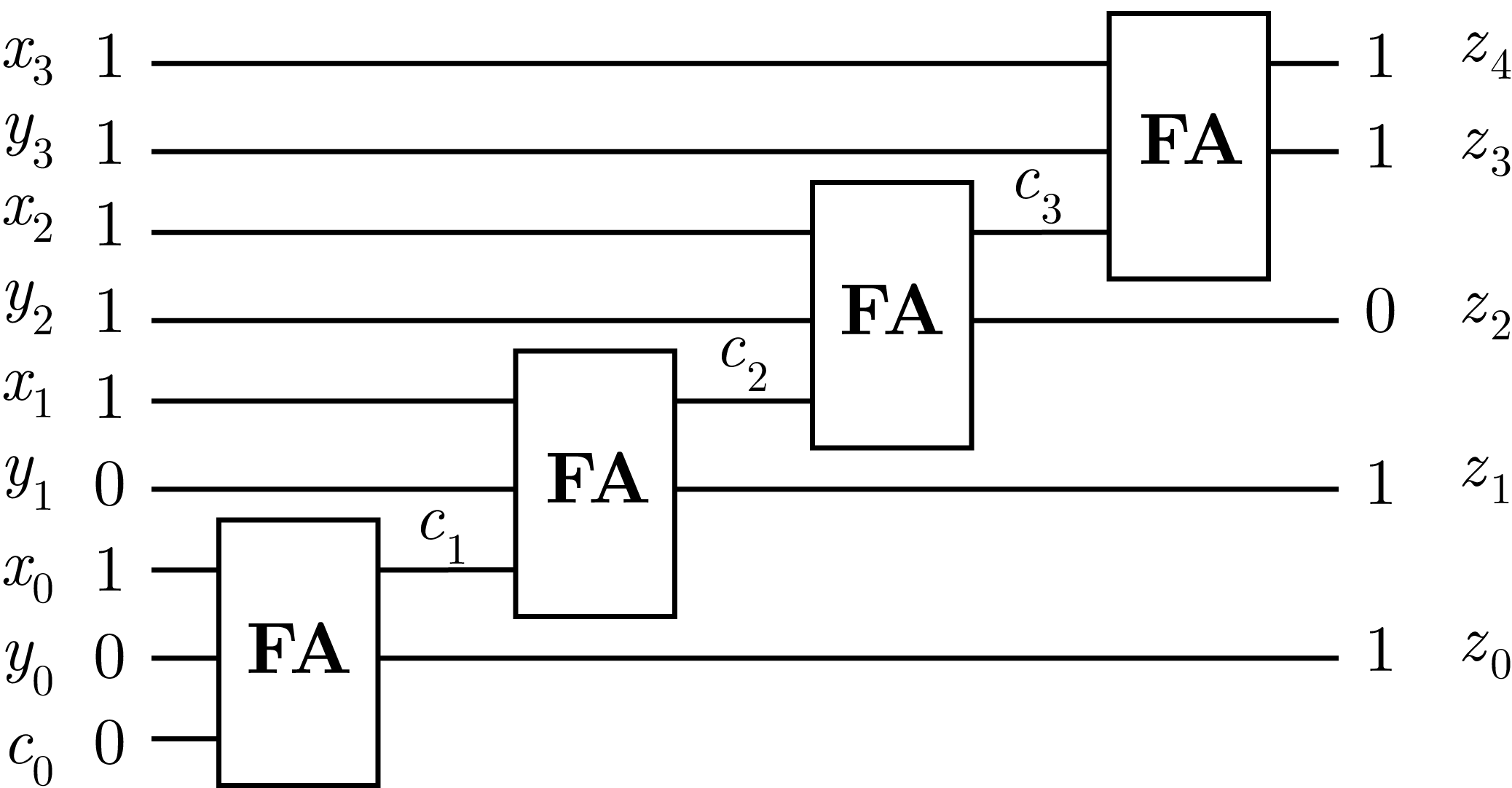The algorithm we've constructed for addition is an example of an algorithm that runs in polynomial time. It requires $\mathcal{O}\big(n^1\big)$ (read as "on the order of $n$") repeated operations.

Some algorithms require $\mathcal{O}\big(n^2\big)$ or higher polynomial orders of input size $n,$ but all polynomial time algorithms are usually considered efficient compared to algorithms that take exponential time $\mathcal{O}\big(2^n\big).$

# Computing with Circuits

Addition isn't the only arithmetic operation that can be mapped to simple binary logical circuits. In fact, it can be proven that any arbitrary function $f(\mathbf{x})$ can also be mapped to these simple operations. This is how classical computers convert a program written in Python into instructions that the CPU can follow.

Some of these problems are easy — adding two numbers together is a problem that can be solved by a tractable algorithm (an algorithm that runs in polynomial time). But some are hard, like finding the prime factors of a 2048-bit binary number, or finding the optimal fold of a protein.

There are some problems that are very hard to solve, and even though we know how to solve small versions of them with logic circuits, they take a very long time to run. Inefficient algorithms for these hard problems often have exponential time requirements $\mathcal{O}(2^n)$.

Why are exponential time algorithms $\big($even relatively fast ones like $\mathcal{O}({\small2^{n/10}})\big)$ considered intractable while polynomial ones $\big($even slow ones like $\mathcal{O}({\small n^{10}})\big)$ are considered efficient?

# Computing with Circuits

Why did we just spend so much time learning about how a classical computer works?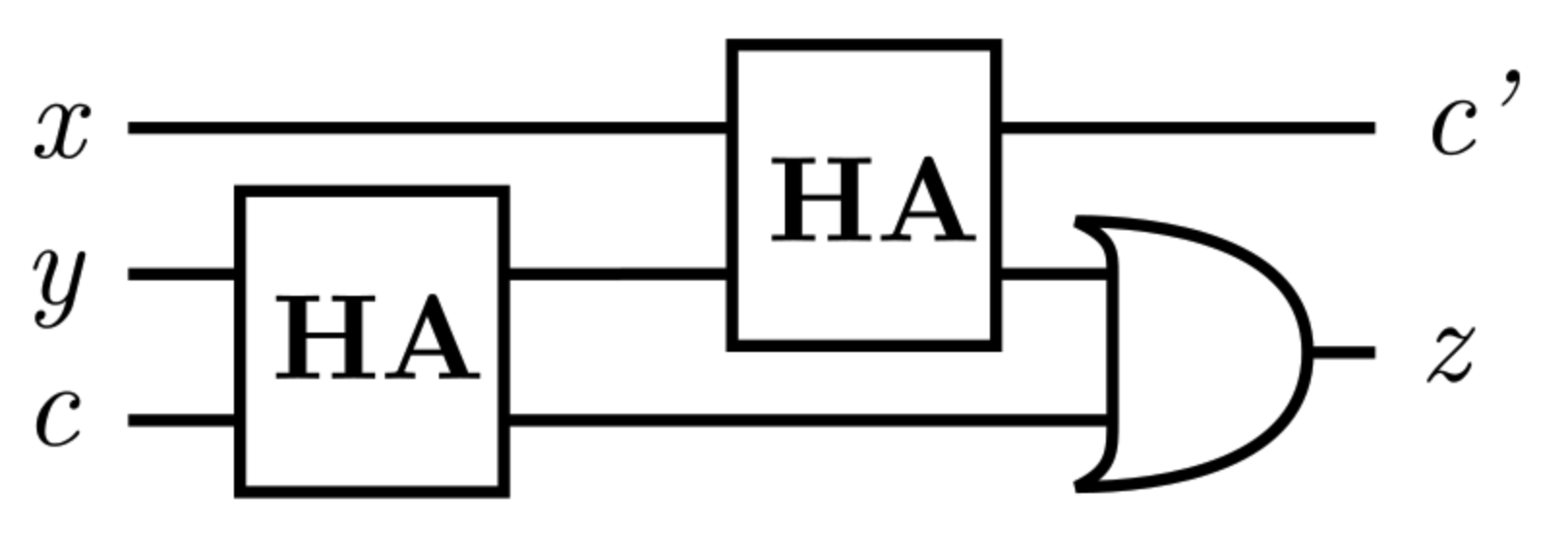Computers and the theories of classical computation were mature for decades before the first quantum computers, and so much of the young field of quantum information is based on the simple logical operation of bits that we've begun to explore here.

The quantum circuits we use in this course will look quite a bit like the adder circuit above, so it's best to get familiar now. You can find a great deal more about classical logic and gates on Brilliant, but for now we will go boldly forward into new territory.

Prepare to leave the bit behind, and learn to love the qubit.

# Computing with Circuits

×